Ex 6.3

Chapter 6 Class 12 Application of Derivatives
Serial order wise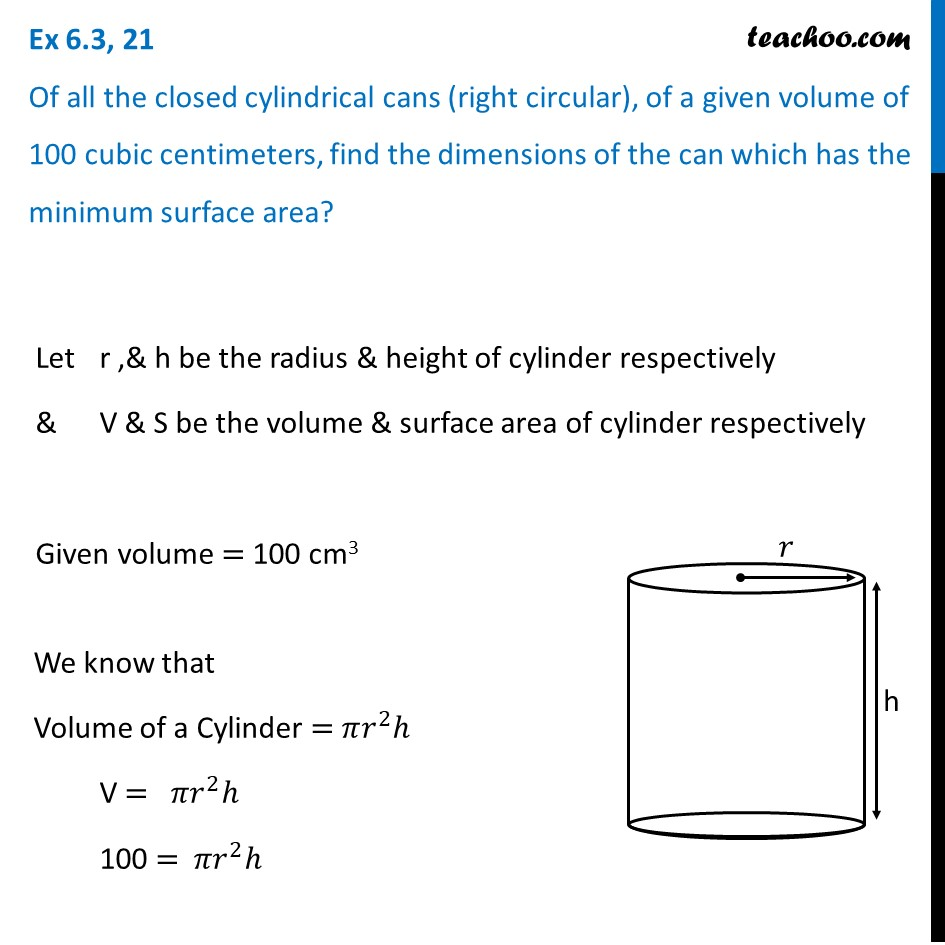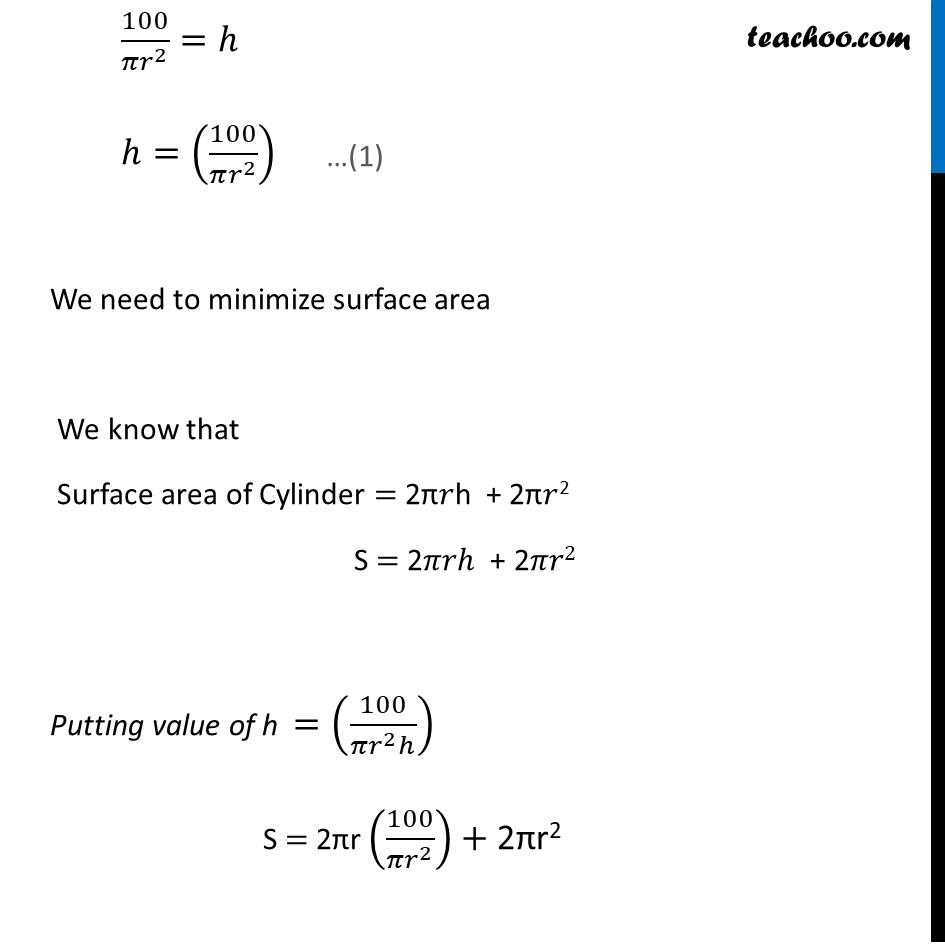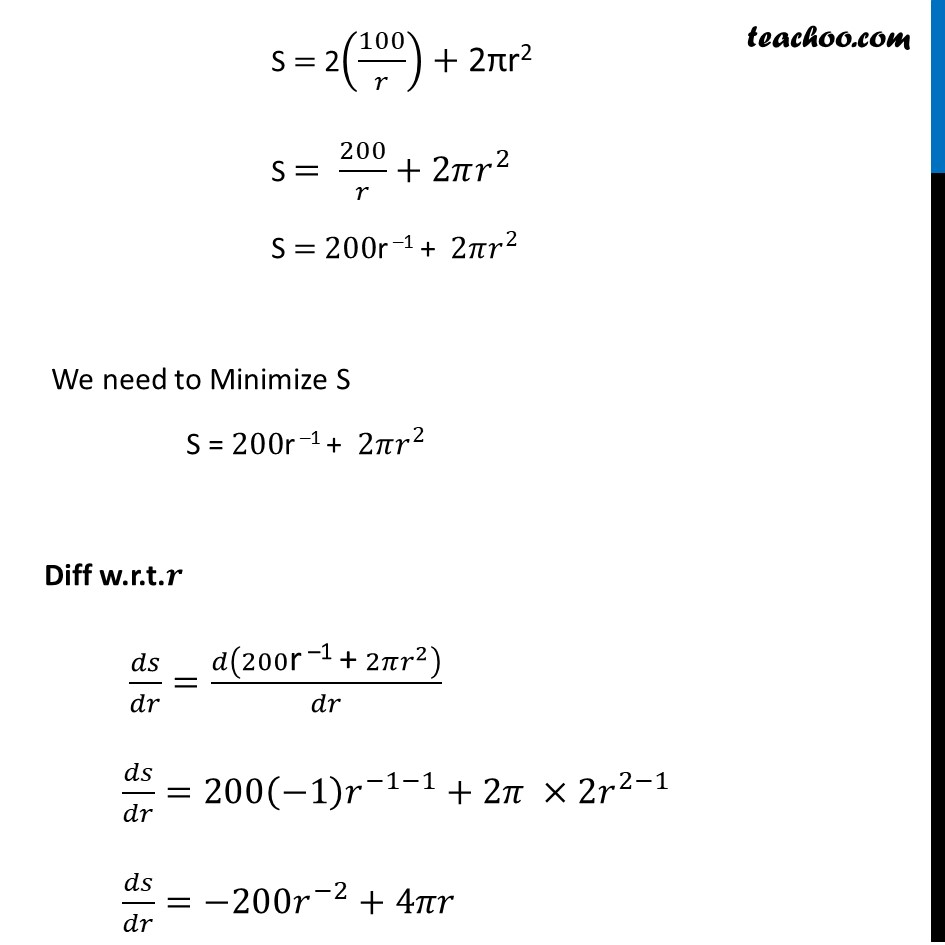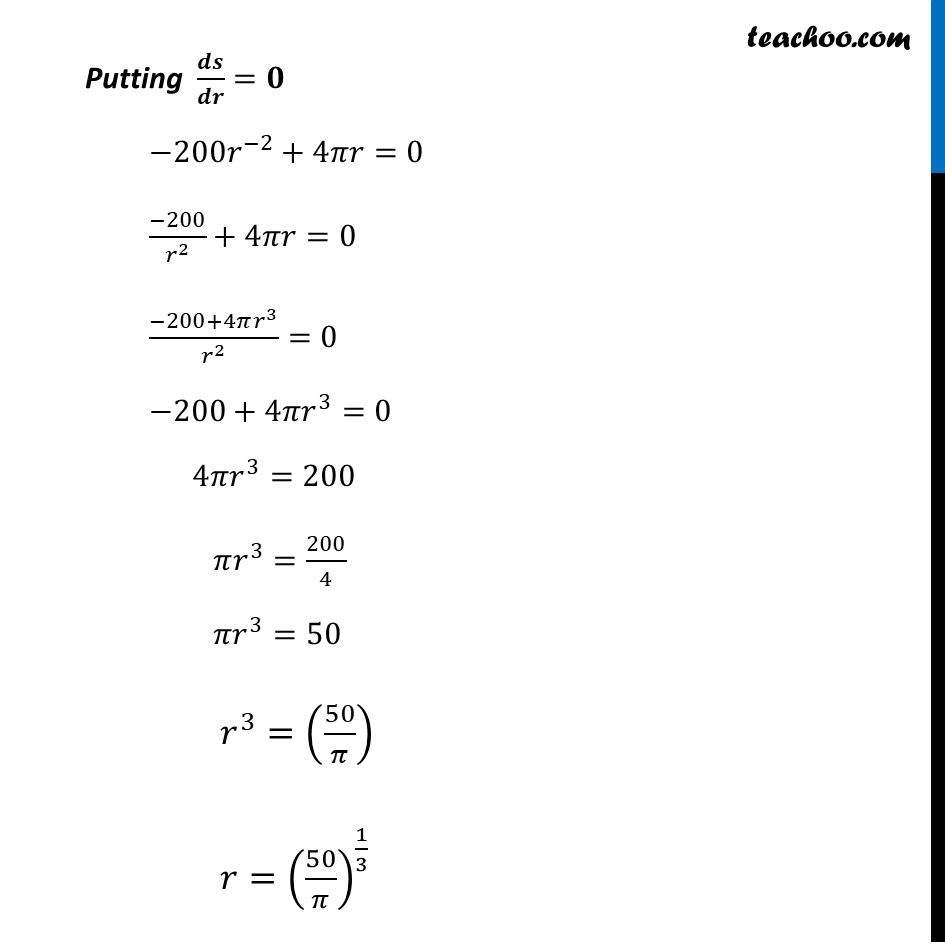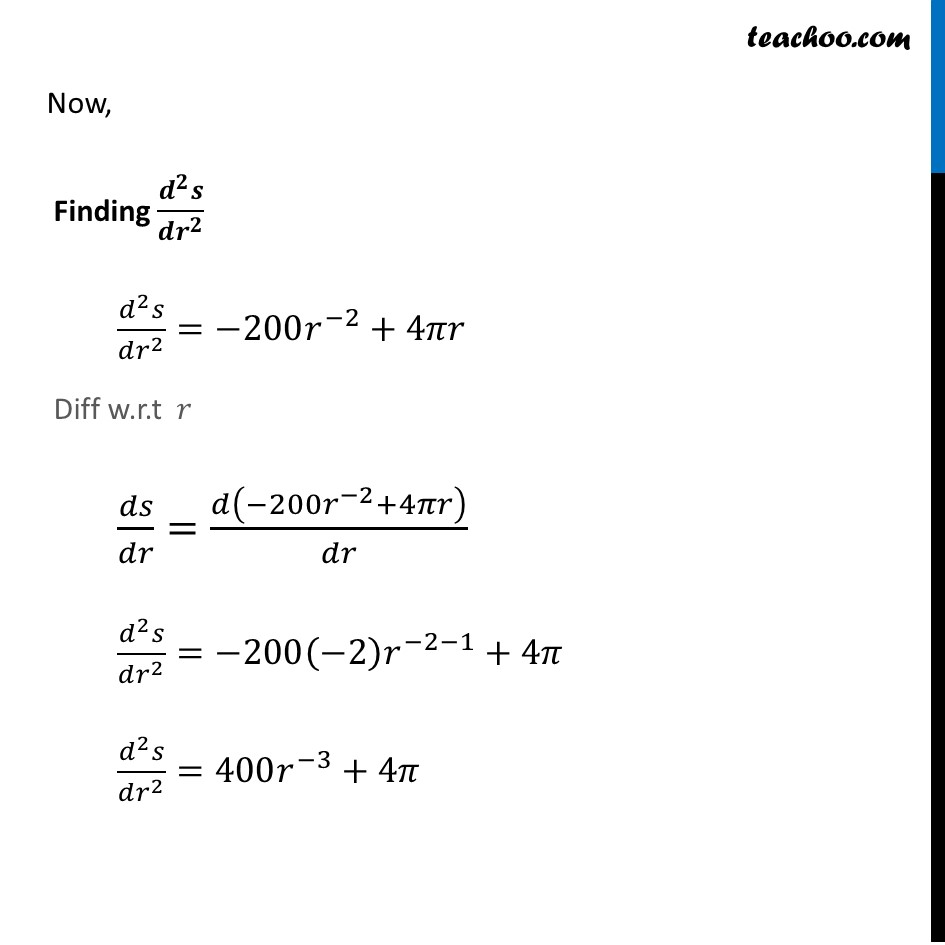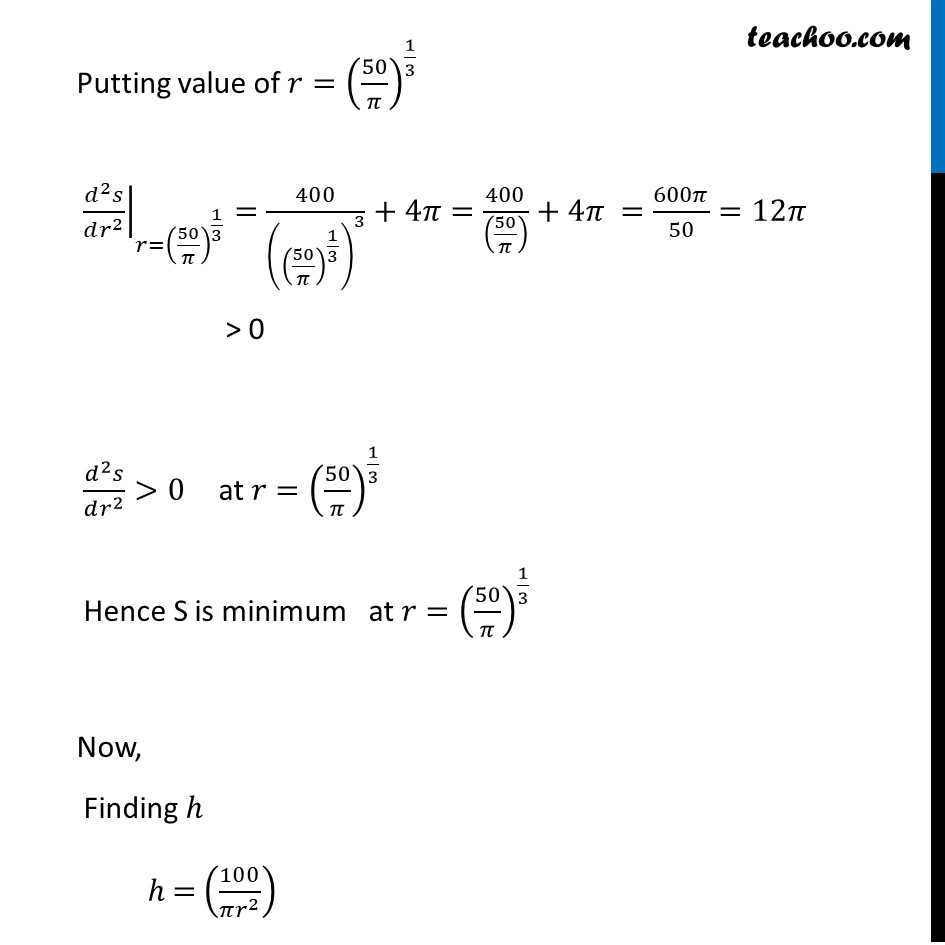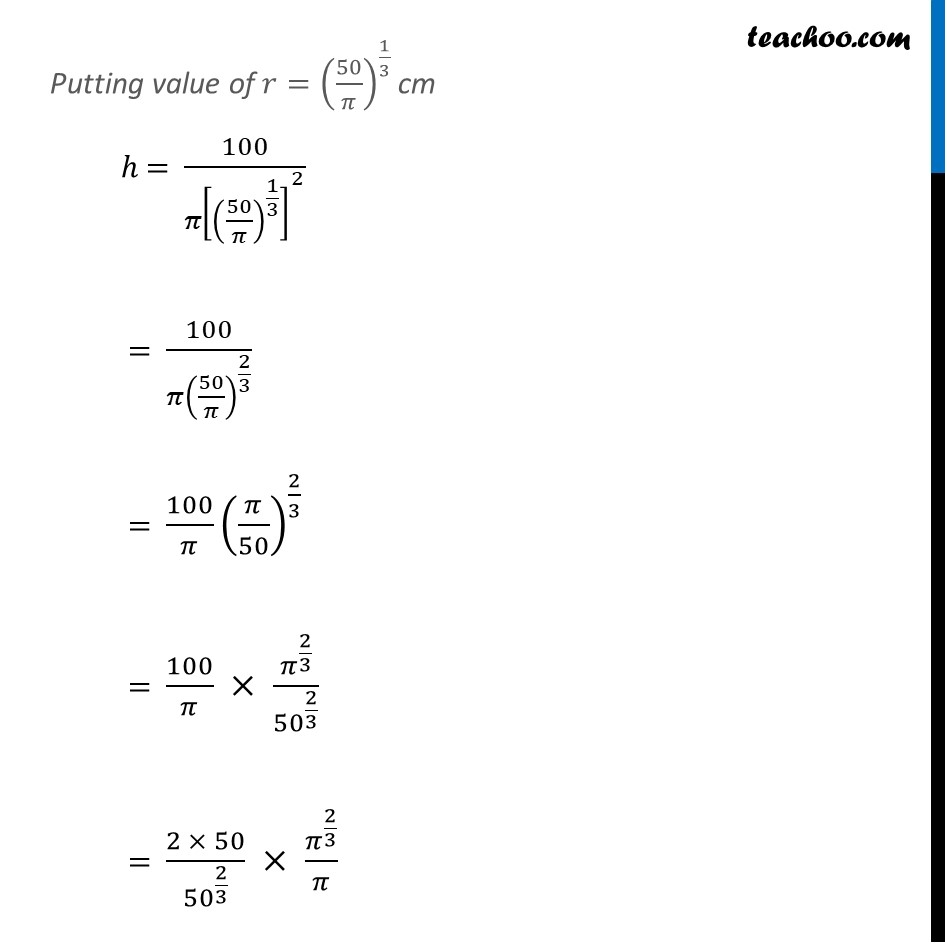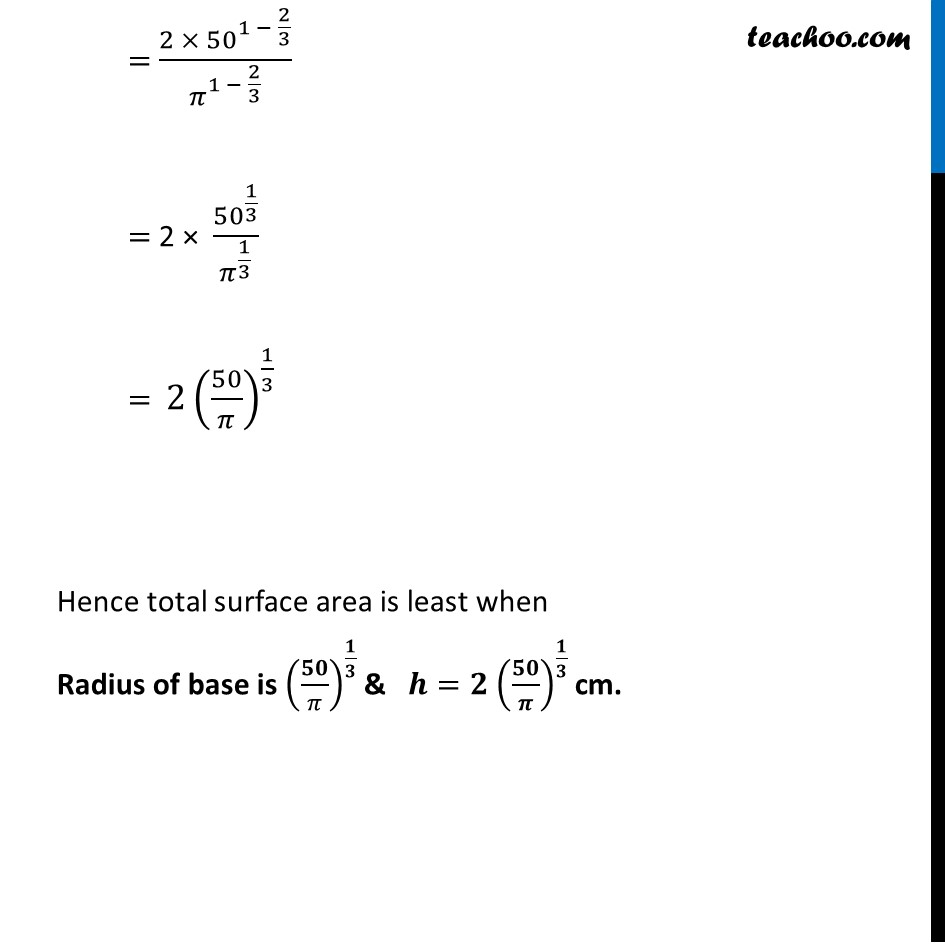Learn in your speed, with individual attention - Teachoo Maths 1-on-1 Class

### Transcript

Ex 6.3, 21 Of all the closed cylindrical cans (right circular), of a given volume of 100 cubic centimeters, find the dimensions of the can which has the minimum surface area? Let r ,& h be the radius & height of cylinder respectively & V & S be the volume & surface area of cylinder respectively Given volume = 100 cm3 We know that Volume of a Cylinder = 𝜋𝑟^2 ℎ V = 𝜋𝑟^2 ℎ 100 = 𝜋𝑟^2 ℎ 100/(𝜋𝑟^2 )=ℎ ℎ=(100/(𝜋𝑟^2 )) We need to minimize surface area We know that Surface area of Cylinder = 2π𝑟h + 2π𝑟2 S = 2𝜋𝑟ℎ + 2𝜋𝑟2 Putting value of h =(100/(𝜋𝑟^2 ℎ)) S = 2πr (100/(𝜋𝑟^2 ))+"2πr2 " S = 2(100/𝑟)+"2πr2 " S = 200/𝑟+2𝜋𝑟^2 S = 200r –1 + 2𝜋𝑟^2 We need to Minimize S S = 200r –1 + 2𝜋𝑟^2 Diff w.r.t.𝒓 𝑑𝑠/𝑑𝑟=𝑑(200"r –1 + " 2𝜋𝑟^2 )/𝑑𝑟 𝑑𝑠/𝑑𝑟=200(−1) 𝑟^(−1−1)+2𝜋 ×2𝑟^(2−1) 𝑑𝑠/𝑑𝑟=−200𝑟^(−2)+4𝜋𝑟 Putting 𝒅𝒔/𝒅𝒓=𝟎 −200𝑟^(−2)+4𝜋𝑟=0 (−200)/𝑟^2 +4𝜋𝑟=0 (−200+4𝜋𝑟^3)/𝑟^2 =0 −200+4𝜋𝑟^3=0 4𝜋𝑟^3=200 𝜋𝑟^3=200/4 〖𝜋𝑟〗^3=50 𝑟^3=(50/𝜋) 𝑟=(50/𝜋)^(1/3) Now, Finding (𝒅^𝟐 𝒔)/(𝒅𝒓^𝟐 ) (𝑑^2 𝑠)/(𝑑𝑟^2 )=−200𝑟^(−2)+4𝜋𝑟 Diff w.r.t 𝑟 𝑑𝑠/𝑑𝑟=𝑑(−200𝑟^(−2)+4𝜋𝑟)/𝑑𝑟 (𝑑^2 𝑠)/(𝑑𝑟^2 )=−200(−2) 𝑟^(−2−1)+4𝜋 (𝑑^2 𝑠)/(𝑑𝑟^2 )=400𝑟^(−3)+4𝜋 Putting value of 𝑟=(50/𝜋)^(1/3) ├ (𝑑^2 𝑠)/(𝑑𝑟^2 )┤|_(𝑟=(50/𝜋)^(1/3) )=400/((50/𝜋)^(1/3) )^3 +4𝜋=400/((50/𝜋) )+4𝜋 =600𝜋/50=12𝜋 > 0 (𝑑^2 𝑠)/(𝑑𝑟^2 )>0 at 𝑟=(50/𝜋)^(1/3) Hence S is minimum at 𝑟=(50/𝜋)^(1/3) Now, Finding ℎ ℎ = (100/(𝜋𝑟^2 )) Putting value of 𝑟=(50/𝜋)^(1/3) cm ℎ = 100/〖𝜋[(50/𝜋)^(1/3) ]〗^2 = 100/〖𝜋(50/𝜋)〗^(2/3) = 100/𝜋 (𝜋/50)^(2/3) = 100/𝜋 × 𝜋^(2/3)/50^(2/3) = (2 × 50)/50^(2/3) × 𝜋^(2/3)/𝜋 = (2 × 50^(1 − 2/3))/𝜋^(1 − 2/3) = 2 × 50^(1/3)/𝜋^(1/3) = 2(50/𝜋)^(1/3) Hence total surface area is least when Radius of base is (𝟓𝟎/𝜋)^(𝟏/𝟑) & 𝒉=𝟐 (𝟓𝟎/𝝅)^(𝟏/𝟑) cm.